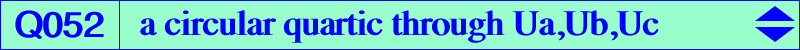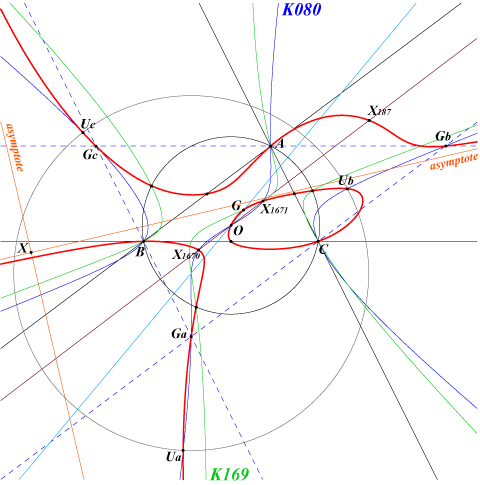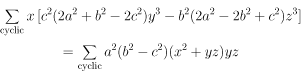X(2), X(3), X(187), X(1670), X(1671), X(3413), X(3414) Ga, Gb, Gc vertices of the antimedial triangle midpoints of ABC points Ua, Ub, Uc mentioned in the Neuberg cubic page. See also table 16 and table 18. X(3413), X(3414) : points at infinity of the Kiepert hyperbolaQ052 is a circular quartic with singular focus X(187) which passes through the points Ua, Ub, Uc. It has two real asymptotes parallel to those of the Kiepert hyperbola intersecting at X = X(15301), a point on the lines X(99)X(187), X(543)X(625), etc. It meets the circumcircle at the same points as K169 = pK(X6, X69).